1. Applications of Kirchhoff’s Laws

While dealing with this law, it is important to note that Law of Conservation of energy and Law of charge are to be taken in a combined format.

The basic areas where this law is applied are:

• There is the nodal voltage method
• There is also the mesh current method that is known as Maxwell format
• The final one is branch-current format

It is important to have knowledge of all these methods.

5.1. Branch-current method

As one of the method that is used for Kirchhoff’s laws, this start off with assuming current that is available at various networks and minimal voltage drops have to be taken into consideration. Also to be noted is that direction in which current is moving is completely arbitrary and there is opposite points that will help in providing the actual value.

It is very important that students have a fair idea of this process since this is not used in present times apart from simple circuits but surely have a great importance in general studies of today.

5.2. Maxwell’s loop (or mesh) current method

This is a format that is generally used for looped areas. Since, most often it is the 2 or 3 mesh loop problems that come in these complex circuits, it is important to check out what the procedure of this mesh format is and how it is preferred in comparison to general methods that are applied.

To obtain currents associated with M-mesh, the voltage results of M-mesh voltage is to be taken. So, with help of mesh currents, a number of confluent issues can be solved. Since, this is associated with energy sources rather than mere current sources, therefore a number of complex aspects can be solved easily.

The procedure that is to be followed in this case are, assuming a minimal number of mesh currents that is available and that is linked to basic elements that are present here. Also, a correct format of Kirchhoff’s equation on voltage is to be taken into consideration. So, a summation of these current values are done and states direction of currents.

5.3. Nodal voltage method

This is another method of applying the Kirchhoff’s laws and is used on a regular basis for computer network. In this case it has to be noted that this branch voltage is noted at vend of each of these ends of a terminal and in case of conductor’s attachment with anode.

A situation that has to be taken in this case is assumption has to be made of different voltages that occur at different nodal points. So in spite of having a number of independent circuit points, a summation of all has to be done in equation format to get the answer.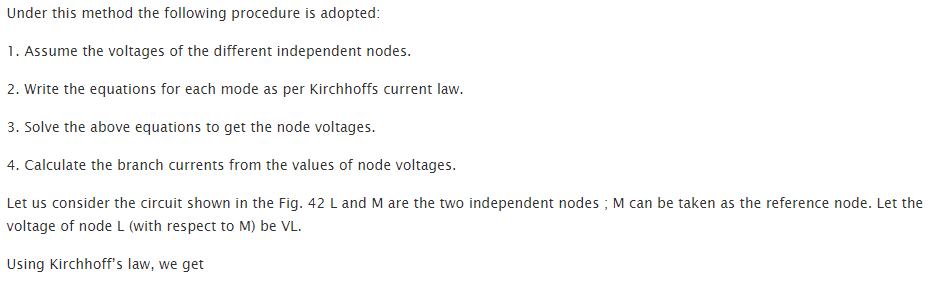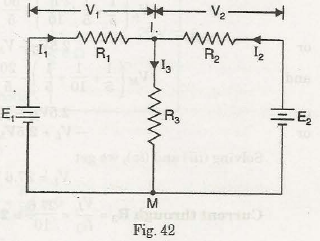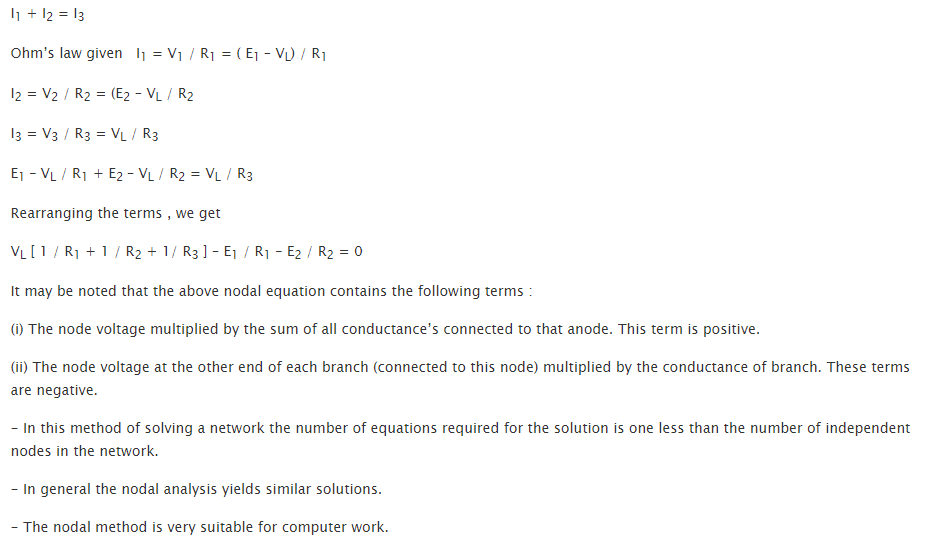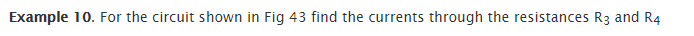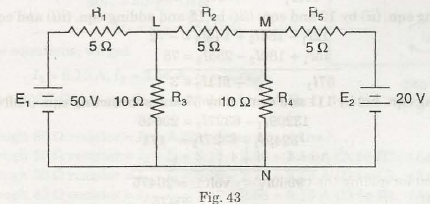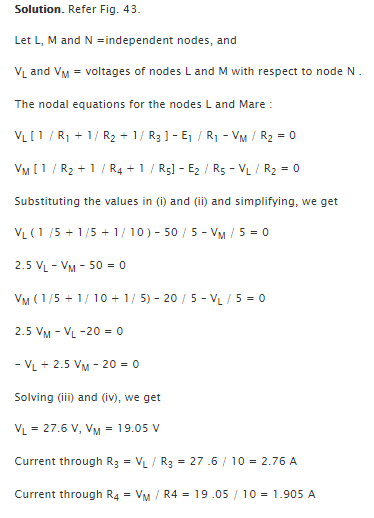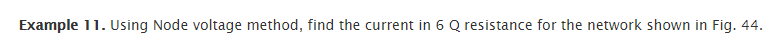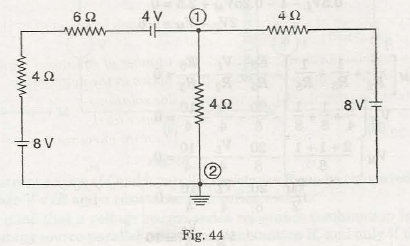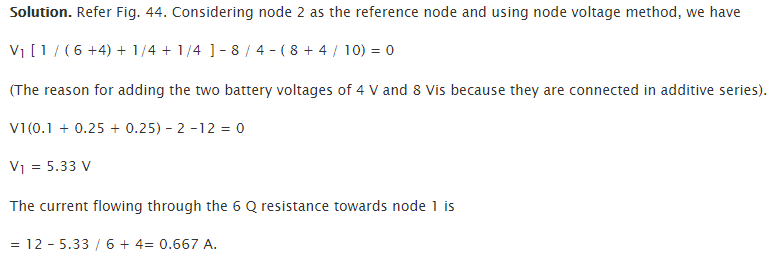Links of Next Electrical Engineering Topics:-### Customer Reviews

My Homework Help
Rated 5.0 out of 5 based on 510 customer reviews at